U.S. Department of Transportation
1200 New Jersey Avenue, SE
Washington, DC 20590
202-366-4000

Federal Highway Administration Research and Technology
Coordinating, Developing, and Delivering Highway Transportation InnovationsThis report is an archived publication and may contain dated technical, contact, and link information
 Federal Highway Administration > Publications > Research Publications > 05137 > Evaluation of Safety, Design, and Operation of Shared-Use Paths
 Publication Number: FHWA-HRT-05-137 Date: July 2006
`

# Evaluation of Safety, Design, and Operation of Shared-Use Paths

## Final Report

PDF files can be viewed with the Acrobat® Reader®

## CHAPTER 3. DEVELOPMENT OF THEORY

### INTRODUCTION

This chapter discusses the theoretical underpinnings of the proposed operational models. The first model (discussed in the next section) was developed for the purpose of estimating the desired number of active passing, passive passing, and meeting events on a shared path from the perspective of the bicyclist. In calculating the desired number of events, it is assumed that the path has adequate width, so that no passing or other events are constrained by the path geometry. Active passing refers to the situation where the average bicyclist (traveling at the average bicycle speed) desires to pass slower moving vehicles on the path (i.e., bicycles, pedestrians, inline skaters, etc.). Passive passing refers to the average bicyclist being overtaken by faster moving bicyclists or other modes. Meeting refers to the number of opposing vehicles that are met while the average bicyclist is on the path. This model is demand oriented and uses primarily the attributes of the mixed traffic flow that are on the path. These attributes consist of the modal volumes and their respective means and standard deviations of speeds over the path. As will be indicated in chapters 4 and 5 on field data collection and analysis, the modal attributes pertaining to speed are based on the field-verified assumption that speeds are normally distributed with a given mean and standard deviation that may vary from mode to mode.

The second model discussed in this chapter imposes constraints on the number or fraction of passing maneuvers that can be executed because of the geometry of the path. The motivation for focusing on active passing maneuvers is founded on the results of the perception data analysis (chapter 7), where the primary impedance reported is related to the inability of the bicyclist to pass other users on the path. In this model, the path width is converted into an equivalent number of lanes in each direction, and a probabilistic model of the delayed passing maneuvers for each lane configuration is estimated. The LOS method described in chapter 8 uses the actual number of delayed passings per hour (which is the product of the desired passing maneuvers and the probability of a delayed passing) in determining the path LOS.

### ESTIMATING THE NUMBER OF EVENTS

The estimates shown in this chapter are founded on the original work done by Botma(5, 43) and are partially documented in the 2000 HCM.(4) The original work by Botma was confined to two modes, namely pedestrians and bicycles. The proposed model extends Botma's calculations to estimate the desired number of meetings and passings by any vehicle (bicycle, pedestrian, inline skater, etc.) at any desired speed. As stated in the previous section, the speeds of the various modes are normally distributed, and this assumption was verified with field data. A second difference between the proposed approach and Botma's is the numerical nature of the model. This is because the area under the normal distribution curve (representing modal speed) is needed to calculate the estimated number of events. For simpler speed distributions (e.g., uniform distribution), closed-form solutions can be derived. However, because the method is numerical, it should be able to cope with any (or more than one) speed distribution for each of the modes using the path. The same type of models can be used to look at cross-modal meetings and passings (e.g., bicycles passing pedestrians, etc.). The model is meant to be applied for one pair of modes at a time. This means that the procedure first predicts the number of events encountered by the average bicyclist when considering other bicyclists only. The model is then re-applied when considering another mode (e.g., pedestrians), and so forth. The total number of events is then summed across all modes, and provides an overall picture of the multimodal passing and meeting demand on the path.

The following derivations are therefore limited to a pair of modes. The "test unit" in this case refers to the bicycle mode. Modal flow rates and speed apply to the mode that is impeding the bicycle mode on the path.

Glossary of Variables

1. L = Length of path, mi
2. Q = Modal flow rate (units past a point) in subject direction, per hour
3. Q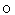= Modal flow rate in opposite direction (units/h)
4.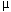= Modal mean speed in subject direction (mi/h)
5.= Modal mean speed in opposite direction (mi/h)
6.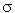= Modal standard deviation of speed in subject direction (mi/h)
7.= Modal standard deviation of speed in opposite direction (mi/h)
8.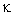= Modal density in subject direction (units/mi)
9.= Modal density in opposite direction (units/mi)
10. N= Number of modal units on the path in subject direction (N = kL)
11. N= Number of modal units on the path in the opposite direction (N= kL)
12. U = Speed of the test unit (mi/h)
13.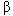Threshold speed ratio for initiating an active passing (default = 1)
14.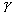= Threshold speed ratio for initiating a passive passing (default = 1)

### ESTIMATING ACTIVE PASSING EVENTS

Active passing is defined as the desired number of passing maneuvers for the test vehicle (in our case, a bicycle, but it could be any mode), which is traveling at a constant speed, U, and encountering an impeding modal stream (other bicycles, pedestrians, etc.), which is traveling in the same direction at a speed (v) that is normally distributed in space with N(,2). This situation is depicted graphically in figure 1.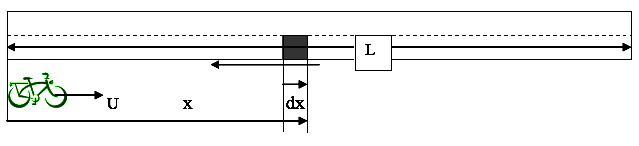Figure 1. Schematic for active passing estimation.

In our example, the test vehicle is a bicycle that is traveling at speed U. Let x be the distance from the location of the test bicycle to a strip on the path of length dx. By definition, the expected number of modal units in dx is k * dx. The desired number of passings by the test bicycle of those units in dx can be calculated as follows. The test bicycle will pass only those units in dx that will exit the segment L after the test bicycle has exited. Mathematically, this is defined as: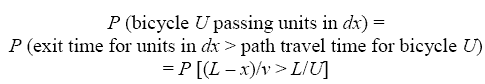(8)

which, with some manipulation, gives: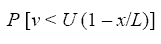(9)

Therefore, the expected number of active passings for strip dx will be: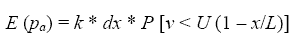(10)

Since v is distributed with N(,2), then the stated probability in equation 10 can be easily calculated from the integral under the standard normal curve. By dividing the full length of the shared path into small discrete slices, each of length dx, the cumulative probability, F(x), and the expected number of active passing maneuvers can be calculated as the average of the probability at the start and end of each slice, as shown in equation 11 below: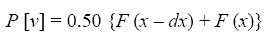(11)

The number of active passing events is then summed over all slices to produce the desired passings for the entire path.

As an illustration, the following numerical example demonstrates the model application:

Let L = 1 mi, Q = 400 bicycles per hour, x = 0.2 mi, dx = 0.01 mi, U = 15.5 mi/h,= 12.5 mi/h, and= 3 mi/h. The density of modal users is estimated at k = 400/12.5 = 32 vehicles/mi (1 mi = 1.61 km, 1 mi/h = 1.61 km/h). Applying equation 11,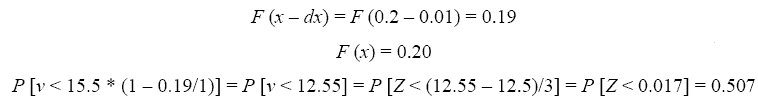(12)

where Z is the standard normal variable. Similiarly, at the downstream end of the slice: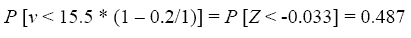(13)

which, according to equation 11, gives: P [ ] = (0.507 + 0.487)/2 = 0.497 and E (pa) = 32 * 0.01 * 0.497 = 0.159 desired passing maneuvers.

By numerically repeating this process for x = 0.01 to 1 mi in increments of 0.01 mi (1 mi = 1.61 km), the total number of active passings on the path can be estimated. For this example, the total number of desired passing maneuvers can be shown to be 6.71 for the test bicycle. A portion of a spreadsheet illustrating the above computations for active passing events is shown in table 5. The above computations are bolded in the table. It should be noted that since the number of passing events is random, the standard deviation is computed as the square root of the mean number of events, based on the Poisson property.

Extension to Exclude Marginal Active Passing Events

The stated probability formulation in the preceding section is quite restrictive in that a desired passing is considered to take place even when there are very small differences in speed between the test unit and slower moving units ahead of it. This assumption can be relaxed to restrict the count of active passing to cases where the ratio of the speed of the passed vehicle to the passing vehicle is below a certain threshold. Let that threshold be b (see table 5). In this case, the probability of passing described in equation 9 can be restated as: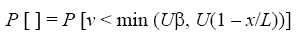(14)

When= 1, the number of active passings estimated by equation 12 reverts to the original formulation in equation 9. As b drops below 1, the probability decreases, and so does the number of desired passings. For the above example, a beta threshold of 0.80 (meaning that a desired passing occurs only if the passed vehicle speed is below 80 percent of the passing vehicle speed) will result in a drop of active passings from 6.71 to 5.66, a reduction of about 16 percent.

Table 5. Computational spreadsheet for active passing events

 X ALPHA V(X) F(VX) F(Vx+DX) F N PASS-ACTIVE Input Output Q = 400 vph Travel time= 3.87 min MU = 12.5 mi/h K = 32 vpm SIGMA = 3 mi/h N = 32 veh U = 15.5 mi/h Mean active passings, path totals = 6.71 L = 1 miles Standard deviation = 2.59 BETA = 1 (<= 1.0) Passing rate per hour = 104 15.50 1 0.01 15.35 0.841 0.829 0.835 0.267 2 0.02 15.19 0.829 0.815 0.822 0.263 3 0.03 15.04 0.815 0.801 0.808 0.259 4 0.04 14.88 0.801 0.786 0.794 0.254 5 0.05 14.73 0.786 0.771 0.779 0.249 6 0.06 14.57 0.771 0.755 0.763 0.244 7 0.07 14.42 0.755 0.738 0.747 0.239 8 0.08 14.26 0.738 0.721 0.730 0.234 9 0.09 14.11 0.721 0.704 0.712 0.228 10 0.10 13.95 0.704 0.686 0.695 0.222 11 0.11 13.80 0.686 0.667 0.676 0.216 12 0.12 13.64 0.667 0.648 0.658 0.210 13 0.13 13.49 0.648 0.629 0.638 0.204 14 0.14 13.33 0.629 0.609 0.619 0.198 15 0.15 13.18 0.609 0.589 0.599 0.192 16 0.16 13.02 0.589 0.569 0.579 0.185 17 0.17 12.87 0.569 0.548 0.559 0.179 18 0.18 12.71 0.548 0.528 0.538 0.172 19 0.19 12.56 0.528 0.507 0.518 0.166 20 0.20 12.40 0.507 0.487 0.497 0.159 21 0.21 12.25 0.487 0.466 0.476 0.152 22 0.22 12.09 0.466 0.446 0.456 0.146 23 0.23 11.94 0.446 0.425 0.435 0.139 24 0.24 11.78 0.425 0.405 0.415 0.133 25 0.25 11.63 0.405 0.385 0.395 0.126 26 0.26 11.47 0.385 0.366 0.375 0.120 27 0.27 11.32 0.366 0.346 0.356 0.114 28 0.28 11.16 0.346 0.328 0.337 0.108 29 0.29 11.01 0.328 0.309 0.318 0.102 1 mi = 1.61 km1 mi/h = 1.61 km/h

Estimating the Number of Passive Passing Events

By definition, passive passing events refer to the number of units that will overtake the test vehicle as it travels at speed U over the path. The test vehicle is overtaken by other units traveling in the same direction at speeds that are characterized by a normal distribution N(,2). Units that are already in the path cannot overtake a test vehicle that is about to enter the path. Passive passing events are thus calculated for units that are about to enter the path, based on their travel time relationship to that of the test unit. Again, a unit will pass the test unit if: (1) it is behind the test unit when the test unit enters the path, and (2) it will exit the path prior to the test unit. This process is illustrated in figure 2 below.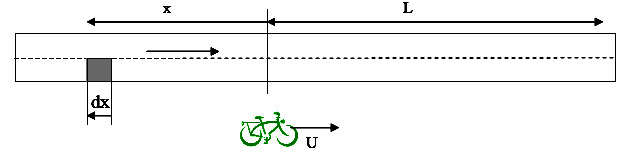Figure 2. Schematic for passilve passing estimation.

In this case, the probability that a modal unit at location dx (e.g., faster bicycle) will pass the test bicycle, which is traveling at speed U while on path L, is the probability that the unit will exit the path prior to the test bicycle, or: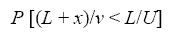(15)

which can be rewritten as: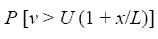(16)

The expected number of passive passings because of the units that are in dx at time zero is: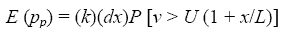(17)

An important question arises regarding the length x needed to capture most of the passive passing events. It is evident that the slower the test unit or the higher the average modal user speed, the longer x should be. Define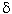as the lowest probability of passing to be included in the computations. Note that as x increases, the probability of a passive passing event decreases. Then, the objective is to find the lowest x, x*, such that: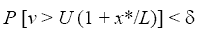(18)

Assuming a normal distribution of speeds over space, the standard normal variable Z that corresponds tois Zand P (Z > Z) =. Then, the minimum value of x is computed as: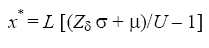(19)

As an example, let L = 1 mi, x = 0.2 mi, dx = 0.01 mi, Q = 400 vehicles per hour,= 12.5, s= 3, U = 9.5 mi/h, and= 0.01 (1 mi = 1.61 km, 1 mi/h = 1.61 km/h). From a standard normal distribution table, Z0.01 = +2.326. Substituting into equation 19 gives: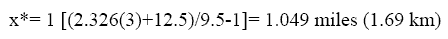(20)

This is slightly longer than the actual path length. If a more accurate estimate is needed-say only probabilities less than 0.005 are ignored-this will require a longer analysis length of 1.8 km (1.129 mi).

Similar to the active passing procedure, the individual slice passings are aggregated over each dx and summed over L to calculate the total number of passive passings on the path. For the example above, it can be shown that for x = 0.20 mi (0.32 km):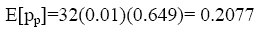(21)

and the total passive passing events over the entire path are estimated at 10.95. Portions of a computational spreadsheet that executes the above equations are shown in table 6.

Table 6. Computational spreadsheet for passive passing events.

Input   Output
Q = 400 vph Travel time= 6.316 min
MU = 12.5 mi/h K = 32 vpm
SIGMA = 3 mi/h N = 32 vpm
U = 9.5 mi/h X minimum = 1.049 miles
X = 1 miles Mean passive passings,
path totals =
10.95
GAMMA = 1 (>=1) Standard deviation = 3.31
DELTA = 0.01   Passing rate per hour = 104
X ALPHA V(X) F(VX) F(Vx+DX) F N PASS-PASSIVE
9.500
1 0.01 9.595 0.841 0.834 0.837 0.268
2 0.02 9.690 0.834 0.826 0.830 0.265
3 0.03 9.785 0.826 0.817 0.821 0.263
4 0.04 9.880 0.817 0.809 0.813 0.260
5 0.05 9.975 0.809 0.800 0.804 0.257
6 0.06 10.070 0.800 0.791 0.796 0.255
7 0.07 10.165 0.791 0.782 0.786 0.252
8 0.08 10.260 0.782 0.772M 0.777 0.249
9 0.09 10.355 0.772 0.763 0.768 0.246
10 0.10 10.450 0.763 0.753 0.758 0.242
11 0.11 10.545 0.753 0.743 0.748 0.239
12 0.12 10.640 0.743 0.732 0.738 0.236
13 0.13 10.735 0.732 0.722 0.727 0.233
14 0.14 10.830 0.722 0.711 0.716 0.229
15 0.15 10.925 0.711 0.700 0.706 0.226
16 0.16 11.020 0.700 0.689 0.695 0.222
17 0.17 11.115 0.689 0.678 0.683 0.219
18 0.18 11.210 0.678 0.666 0.672 0.215
19 0.19 11.305 0.666 0.655 0.661 0.211
20 0.20 11.400 0.655 0.643 0.649 0.208
21 0.21 11.495 0.643 0.631 0.637 0.204
22 0.22 11.590 0.631 0.619 0.625 0.200
23 0.23 11.685 0.619 0.607 0.613 0.196
24 0.24 11.780 0.607 0.595 0.601 0.192
25 0.25 11.875 0.595 0.583 0.589 0.188
26 0.26 11.970 0.583 0.570 0.576 0.184
27 0.27 12.065 0.570 0.558 0.564 0.180
28 0.28 12.160 0.558 0.545 0.551 0.176
29 0.29 12.255 0.545 0.533 0.539 0.172
1 mi = 1.61 km
1 mi/h = 1.61 km/h

Extension to Exclude Marginal Passive Passing Events

Similar to the active passing condition, the above formulation can be extended to restrict passive passing to cases where the ratio of the speed of the passing vehicle to the speed of the passed vehicle is above a certain threshold. Let that threshold be; therefore, the probability of passing can be restated as: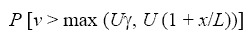(22)

For= 1, the number of passive passings in equation 18 reverts to the original formulation (equation 16). Asincreases above 1, the probability decreases, and so does the number of desired passings. For the above example, a Gamma threshold of 1.25 (meaning that a desired passing occurs only if the passing vehicle speed is 25 percent above the slower vehicle speed) will result in a drop of active passings from 10.95 to 9.96 (about 9.1 percent).

### Estimating the Number of Meetings

Using the same concepts outlined in the previous section for passive passing events, let x be defined as a segment of the path upstream from the segment of interest in the opposing direction. It is obvious from the schematic in figure 3 that every opposing vehicle that is present on the path when the test unit enters it will meet the test unit regardless of speed, assuming, of course, that no vehicle enters or exits the path at an intermediate point. In addition, all opposing vehicles that enter the segment before the test vehicle exits it will also meet the test unit. The computations in this section focus on this second term.

The probability of a meeting because of units that are present at location dx at time zero (when the test vehicle enters the path) is estimated as: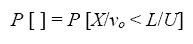(23)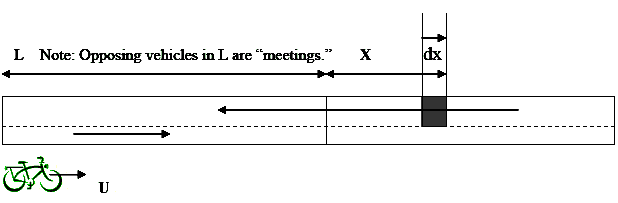Figure 3. Schematic for meeting event estimation.

Which can be reorganized as: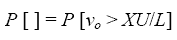(24)

and the corresponding average number of meeting events because of units in dx is: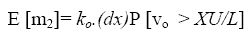(25)

Similar to the case of passive passings, the minimum length x* to guarantee a minimum probability d of inclusion into the computations of meetings can be calculated from the equation: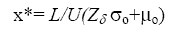(26)

where Z is the standard normal variable. The total number of meetings is then calculated as the sum of the meetings with opposing vehicles that are already on the path when the test unit enters it (this is simply the equivalent of the opposing density times the path length) and meeting with vehicles that have yet to enter the path (as described by equation 25). Therefore, the total meeting events are computed as: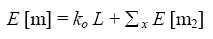(27)

For a numerical example of the calculation of the number of meetings, assume that there is a vehicle positioned between x = 0.19 mi and x = 0.20 mi when the test bicycle enters the path. Also assume L = 1 mi, x = 0.20 mi, dx = 0.01 mi, U = 15.5 mi/h, Q= 400 vehicles per hour,= 12.5 mi/h,= 3 mi/h, and d = 0.01 (1 mi = 1.61 km, 1 mi/h = 1.61 km/h). We first determine the minimum virtual path length needed to capture 99 percent of all meetings. From equation 26: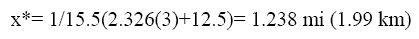(28)

so that 124 segments, each 0.01 mi in length, would be required to numerically compute the meetings. From equation 24: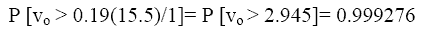(29)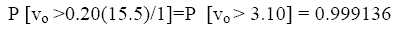(30)

so that P[0.19 - 0.20] = (0.9993 + 0.999276)/2 = 0.999206. The expected number of meetings per equation 25 is computed as: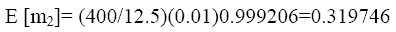(31)

Finally, the total expected number of meetings per equation 27 is: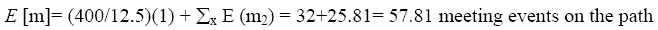(32)

A partial computational spreadsheet that executes the above equations for estimating the number of meeting events is shown in figure 6. For comparison purposes, table 7 contrasts the numerical estimates of meetings developed in this work with Hein Botma's(5) analytical estimate given in equation 33: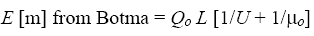(33)

In most cases (assuming the minimum x value is satisfied), the two estimates are identical.

Table 7. Computational spreadsheet for meeting events.

Input   Output
Q = 400 vph Travel time= 3.87 min
MU = 12.5 mi/h Ko = 32 vpm
SIGMA = 3 mi/h No = 32 veh
U = 9.5 mi/h X minimum = 1.2566 miles
X = 1 miles Average meetings, path totals = 57.81
DELTA = 0.01   Standard deviation = 7.60
Botma's analytical estimate = 57.81
Meeting rate per hour = 896

X ALPHA V(X) F(VX) F(Vx+DX) F N MEETINGS
0.000       32.000 on path
1 0.01 0.155 1.000 1.000 1.000 0.320
2 0.02 0.310 1.000 1.000 1.000 0.320
3 0.03 0.465 1.000 1.000 1.000 0.320
4 0.04 0.620 1.000 1.000 1.000 0.320
5 0.05 0.775 1.000 1.000 1.000 0.320
6 0.06 0.930 1.000 1.000 1.000 0.320
7 0.07 1.085 1.000 1.000 1.000 0.320
8 0.08 1.240 1.000 1.000 1.000 0.320
9 0.09 1.395 1.000 1.000 1.000 0.320
10 0.10 1.550 1.000 1.000 1.000 0.320
11 0.11 1.705 1.000 1.000 1.000 0.320
12 0.12 1.860 1.000 1.000 1.000 0.320
13 0.13 2.015 1.000 1.000 1.000 0.320
14 0.14 2.170 1.000 1.000 1.000 0.320
15 0.15 2.325 1.000 1.000 1.000 0.320
16 0.16 2.480 1.000 1.000 1.000 0.320
17 0.17 2.635 1.000 0.999 1.000 0.320
18 0.18 2.790 0.999 0.999 0.999 0.320
19 0.19 2.945 0.999 0.999 0.999 0.320
20 0.20 3.100 0.999 0.999 0.999 0.320
21 0.21 3.255 0.999 0.999 0.999 0.320
22 0.22 3.410 0.999 0.999 0.999 0.320
23 0.23 3.565 0.999 0.999 0.999 0.320
24 0.24 3.720 0.999 0.998 0.998 0.319
25 0.25 3.875 0.998 0.998 0.998 0.319
26 0.26 4.030 0.998 0.998 0.998 0.319
27 0.27 4.185 0.998 0.997 0.997 0.319
28 0.28 4.340 0.997 0.997 0.997 0.319
29 0.29 4.495 0.997 0.996 0.996 0.319
1 mi = 1.61 km
1 mi/h = 1.61 km/h

Sensitivity of Passing Events to Key Parameters

In this section, we present the results of a limited sensitivity analysis of the rate of desired passing events. We conducted two experiments in which the speed of the test unit and the directional flow rate, respectively, were allowed to vary while keeping all other parameters fixed. These analyses were important as a first step in understanding the basis for setting LOS thresholds for shared paths. In both experiments, the following parameters were kept fixed: stream speed = 20.1 km/h (12.5 mi/h), standard deviation of speed = 4.8 km/h (3 mi/h), and path travel time = 1 h (to obtain hourly passing rates).

In the first experiment, the test bicyclist speed was allowed to vary from 15.3 to 24.9 km/h (9.5 to 15.5 mi/h) in 1.6-km/h (1-mi/h) increments. This was done to compare the passing requirements for bicyclists on either end of the speed distribution. The results are summarized in figure 7. As expected, active passing demand increased with the test bicyclist speed, while passive passing demand decreased. For a test bicyclist traveling at the average stream speed (20.1 km/h (12.5 mi/h) in the figure), the number of active and passive passing events is exactly the same, as expected. It is important to note from the figure that the total lowest passing demand occurs at that point as well. In other words, the application of the model will always assume the case of the average bicyclist.

It should be remembered, however, that trip purpose could have an impact on which test bicyclist speed to use. It is obvious, for example, that based on the trend in figure 4, those cyclists at either end of the speed spectrum will have to contend with many more events than the average bicyclist does. Not shown are the effects of speed on meetings. These events are much more common, ranging from a low of 704 meetings per hour at a speed of 15.3 km/h (9.5 mi/h) to 896 meetings per hour at a speed of 24.9 km/h (15.5 mi/h), an increase of 28.5 percent. The effect on impeding bicycles in the opposite direction should be considered minimal, and is discussed further in chapter 7.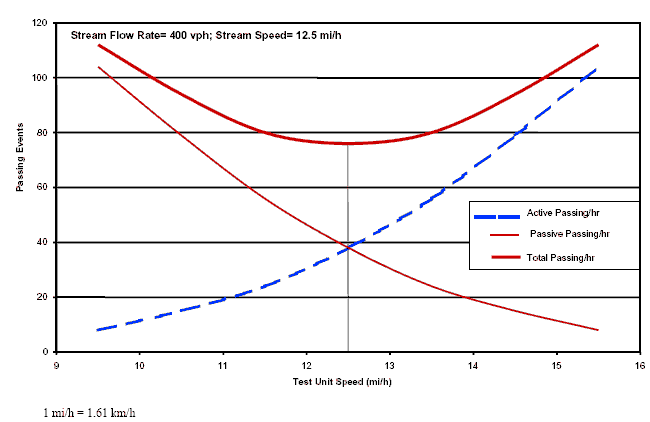Figure 4. Sensitivity of hourly passing rates to individual bicyclist speed.

In the second experiment, the directional flow rate was allowed to vary from 100 to 700 vehicles per hour, in 150-vehicle per hour increments. The test bicyclist speed was fixed at 25 km/h (15.5 mi/h). The results are summarized in figure 5. As expected, all passing rates increased as flow rate increased, with the trend close to linear. It should also be noted that the rate of passive passing events is low given that the test bicyclist speed is, on average, 4.8 km/h (3 mi/h) higher than the average traffic stream speed. On the other hand, the active passing events are quite frequent. The analysis indicates that at an exclusive bicycle path operating with 700 vehicles per hour per direction in which one would like to maintain a speed of 25 km/h (15.5 mi/h) requires an active passing maneuver on average every 3,600/196 = 18 s (obviously a very intolerable situation). By comparison, such maneuvers will occur very infrequently (every 105 s or so) when directional flow is 100 vehicles per hour.

In conclusion, the preceding analysis established a mechanism for identifying passing and meeting event demand rates under a variety of operating conditions. However, the analysis did not account for the supply or capacity side of the path, in particular, how the width of the path or the number of lanes impact the ability to carry out the desired meeting and passing maneuvers. This is explained next, and provides a fundamental approach to determining LOS on multimodal shared-use paths.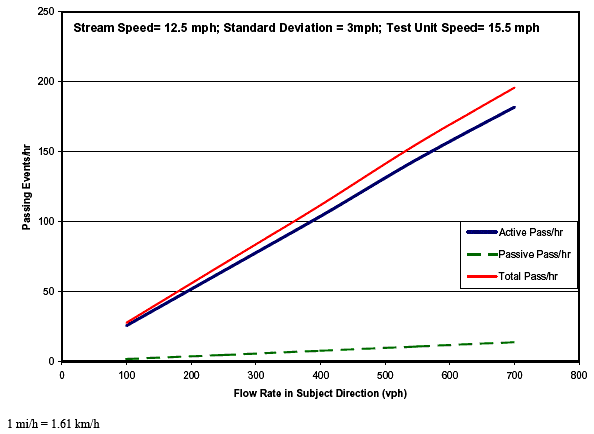Figure 5. Sensitivity of hourly passing rates to directional flow rates.

### ESTIMATING THE PROBABILITY OF DELAYED PASSING

The preceding section focused on estimating the demand for passing maneuvers. In this section, constraints on performing such maneuvers are introduced in the form of delayed passing probabilities. These constraints depend heavily on the geometry of the path, and are analyzed for two-, three-, and four-lane path configurations. There is no attempt to precisely translate width into the number of lanes because much of that translation really depends on how the units actually use the path. However, there are some clear assignments. A 2.4-m- (8-ft-) wide path should always be analyzed as a two-lane path, a 3.6-m (12-ft) path as a three-lane path, and a 4.8-m (16-ft) path as a four-lane path. Intermediate widths, however, are much more difficult to gauge, and the number of effective lanes in those cases will depend very much on the actual use.

In general, and irrespective of configuration, a delayed passing maneuver emerges when there is a bicycle or pedestrian ahead of the overtaking bicycle in the subject direction in conjunction with another opposing bicycle(s) or pedestrian(s) in the opposing direction. This presence of impeding vehicles is computed for a "required passing distance" that is dependent on the type of passing vehicle and type of passed vehicle. For example, chapter 5 shows that a bicycle passing another bicycle requires 33.5 m (110 ft) of clear space, on average, to pass, while only 20.7 m (68 ft) are required for a bicycle to pass a pedestrian. For multi-lane paths (three or more lanes), the procedure below accounts for multiple user groups that may block multiple lanes. Each path configuration is treated separately. Common calibration features are also highlighted as needed.

Glossary of Variables (1 mi = 1.61 km, 1 mi/h = 1.61 km/h)

1. d = Index of a delayed passing maneuver
2. s = Index for the subject direction
3. o = Index for the opposing direction
4. b = Index for blocking two lanes in one direction
5. n = Index for blocking single lane in one direction
6. X = Distance to complete a passing maneuver (mi)*
7. v = Index for vacant path in one direction
8. P = Probability
9. Q = Flow rate for overtaken vehicles in one direction (vehicles per hour)
10. K = Density for overtaken vehicles in one direction (vehicles per mile)
11. U = Space mean speed for overtaken vehicles in one direction (mi/h)

Delayed Passing on Two-Lane Paths

In this case, a delayed passing in the subject direction occurs when, within the distance required to complete a pass (x*), the passing bicycle encounters: (1) traffic in both directions, each blocking a single lane; or (2) no traffic in the subject direction in conjunction with traffic in the opposing direction that is being overtaken by an opposing bicycle. These two cases are illustrated in figure 6.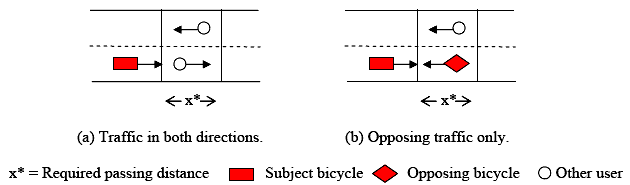Figure 6. Delayed passing cases on a two-lane path.

The delayed passing probability in the subject direction can be expressed as: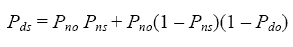(34)

The first term in equation 34 is simply the joint probability of blocking a lane in each of the directions. The other terms are the joint probability of no traffic in the subject lane (1 - P ns), traffic in the opposing lane (Pno), and a nondelayed passing maneuver in the opposing direction (1 - P do).

Similarly, for the opposing direction, it can be shown that: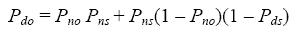(35)

Solving equations 34 and 35 for Pds yields the following expression: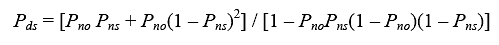(36)

Substituting back into equation 35 yields the corresponding value for Pdo. It is evident from this model that the likelihood of delay for the two-lane case is highly sensitive to the level of opposing traffic.

The above model requires the calibration of two parameters, Pns and Pno. We now assume that the overtaken traffic in the subject direction has a density Ks in vehicles per mile. Thus, for a passing distance x as indicated in figure 9, and assuming Poisson counts, the probability of observing no vehicles over that length (or a vacant segment of length X) is computed as: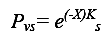(37)

where Ks is estimated as the ratio of flow rate Qs to space mean speed Us for the overtaken traffic in the subject direction. Note that if Ks is computed in units of vehicles per mile, then x in the above equation should be entered in miles as well. Similarly, for the opposing direction: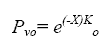(38)

From equations 37 and 38, the probability of having one or more vehicles in segment x is simply computed as: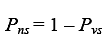(39)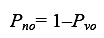(40)

It is important to reiterate that the distance x depends on the mode being passed (i.e., other bicycles, pedestrians, inline skaters, etc.) and is not a constant. Values for x are calibrated from field data and are further explained in chapter 5.

Delayed Passing on Three-Lane Paths

Three-lane paths represent a more difficult challenge for modeling than two-lane paths because the use of the middle lane could create a variety of operational scenarios. To limit the number of possible scenarios, several assumptions were made during the development of the procedure as to how users will behave on the path. The principal three assumptions are:

1. Movements in the subject direction can use the ri.cfmost two lanes, while opposing traffic can use the leftmost two lanes (that means the middle lane can be shared over time).

2. Bicycles passing other vehicles in the same direction must perform that maneuver in the middle lane only; no bicycles are allowed to use the leftmost lane for passing.

3. Groups of users (e.g., pedestrians side by side) can block the two lanes allocated to each direction, but cannot block the leftmost lane allocated to the other direction.

As a result of the above assumptions, the middle lane can be used by:

1. Side-by-side pedestrians, bicyclists, etc., blocking their right and middle lanes.
2. Side-by-side opposing pedestrians, bicyclists, etc., blocking their right and middle lanes, or
3. An opposing bicycle that is overtaking opposing pedestrians, bicyclists, etc., that are blocking their ri.cfmost lane only.

In the three-lane case, a delayed passing in the subject direction occurs when encountering: (a) traffic in the subject direction blocking the ri.cfmost lane only, in conjunction with opposing traffic occupying the other two lanes; or (b) side-by-side users blocking the two ri.cfmost lanes in the subject direction (see assumption 2 above). These cases are illustrated in figure 7. The delay passing probability in the subject direction can be expressed as: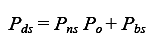(41)

where the probability of opposing traffic occupying the two leftmost lanes is estimated from:(42)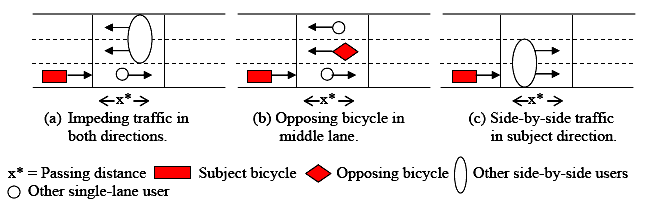Figure 7. Schematic of delayed passing on a three-lane path.

Equation 33 states that opposing traffic occupying the two leftmost lanes can occur in two ways: (1) by having opposing side-by-side users occupying the two lanes (first term of the right side of equation 33), or (2) by having an undelayed opposing passing maneuver (which can occur only if there is an opposing group of users blocking the leftmost lane). Combining equations 41 and 42 gives: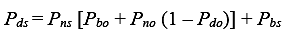(43)

Since the reciprocal case occurs in the opposing direction, it can be shown that: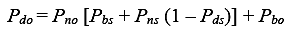(44)

Equations 43 and 44 are simultaneous equations with two unknowns, Pds and Pdo. A closed-form solution can be obtained as follows. First, define: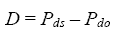(45)

By manipulating equations 44 and 45, it can be shown that: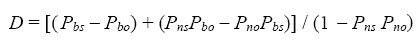(46)

Substituting in equation 43 gives: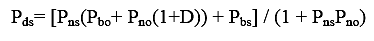(47)

Substituting the value of Pds in equation 38 and D in equation 46 back into equation 45 gives: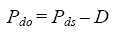(48)

Thus, the above model will estimate the probability of delayed passing in both directions simultaneously. From the above derivation, it is apparent that the model requires the calibration of four probability parameters, two for the subject and two for the opposing directions, namely Pn and Pb in each direction. This is discussed next.

We start by estimating Pb. From field observations of three-lane paths, the fraction of all events in which both lanes are blocked by traffic from the subject direction (case c in figure 10) can be estimated (note that an event is considered to occur only when there is traffic in the subject direction). Let that fraction of events be Fbs. This value is essentially the marginal distribution of Pbs when traffic is present in x. Therefore, we can estimate Pbs in the subject direction as: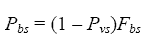(49)

Next, the probability of single-lane blockage Pns is estimated as: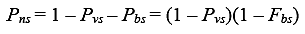(50)

Note that Pvs, the probability of a vacant segment x, is estimated from equation 37 in exactly the same manner as for the two-lane paths. The same procedure is applied for the opposing traffic calibration parameters, yielding the equations below: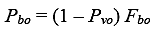(51)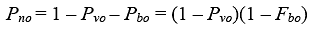(52)

Delayed Passing on Four-Lane Paths

In the case of four-lane paths, the assumption is that the path is divided in a manner similar to a divided four-lane highway. Therefore, the probability of delayed passing is taken to be independent of the amount of opposing traffic since no passing will occur in the two leftmost lanes. This is a conservative assumption, but one that it is necessary to make given the lack of national data on four-lane paths at the volume levels needed to test that assumption. Referring to equation 32, the value of Po is set to zero, and the delayed passing probability is simply equal to Pbs (equation 49 for the subject direction, and Pbo (equation 51) for the opposing direction. Figure 8 depicts the delayed passing concept.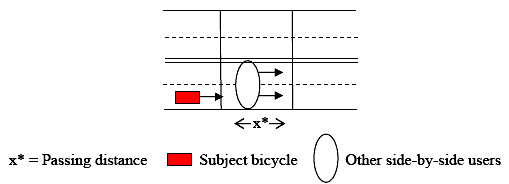Figure 8. Schematic of delayed passingon a four-lane path.

Multimodal Delayed Passing Probability

The probability estimates given in the preceding sections dealt exclusively with one pair of modal users, namely a bicycle passing another mode (other bicycles, pedestrians, etc.). Assuming that the speeds and flows of various modes on the path are independent, the probability of delayed passing under the combination of modes that interfere with the bicycle can be calculated as the probability of no delay from all modes combined. Assuming independence between scenarios (possibly a strong assumption), the aggregate delayed passing probability when encountering m different modes on the path can be shown as: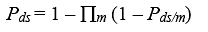(53)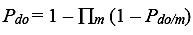(54)

Numerical Application of the Delayed Passing Models

To illustrate the concepts defined in this chapter, a numerical example of a path serving two modes–bicycles and pedestrians–is presented. The data include:

Bicycle Data

• Two-way flow rate (Q) = 500 bicycles per hour.
• Average speed (U) = 20.6 km/h (12.8 mi/h).
• Speed standard deviation = 4.8 km/h (3 mi/h).
• Fraction of bicycle events with bicycles traveling side by side = 0.1.
• Passing distance for bicycle passing bicycle = 32 m (106 ft).
• 50/50 directional split with symmetrical parameters for the opposing flow.

Pedestrian Data

• Two-way flow rate (Q) = 200 pedestrians per hour.
• Average speed (U) = 5.5 km/h (3.4 mi/h).
• Speed standard deviation = 1.6 km/h (1 mi/h).
• Fraction of pedestrians events with pedestrians traveling side by side = 0.35.
• Passing distance for bicycle passing pedestrian = 18 m (60 ft).
• 50/50 directional split with symmetrical parameters for the opposing flow.

The example illustrates the difference in the probability of delayed passing for a two-, three-, or four-lane paths. Since flows and other parameters on the path are fully symmetrical, only the results for the subject direction are reported. Table 8 below summarizes all of the computations by mode and references the source equation for the calculation when applicable.

Table 8. Numerical illustration of path-width effect on delayed passing.

Variables of Interest Two-Lane
Path
Three-Lane
Path
Four-Lane
Path
Bikes Peds. Bikes Peds. Bikes Peds.
Bicycle travel time (min) 4.7 4.7 4.7 4.7 4.7 4.7
Bicycle active passing events 1.83 21.6 1.83 21.6 1.83 21.6
Bicycle active passing rate per hour 23 276 23 276 23 276
Total desired passing rate per hour 299 299 299
Passing distance (Xm) in ft (assumed) 106 60 106 60 106 60
Density (Ksm) vehicles per mile = Q/U 19.5 29.4 19.5 29.4 19.5 29.4
Pvs/m (equation 37) 0.676 0.715 0.676 0.715 0.676 0.715
Fbs/m (assumed) 0.1 0.35 0.1 0.35 0.10 0.35
Pbs/m (equation 49) 0 0 0.033 0.10 0.033 0.10
Pns/m (equations 39 and 50) 0.324 0.285 0.291 0.185 0.291 0.185
Pds/m, % (equations 36, 47, and 49) 26.6 23.6 11.7 14.7 3.3 10.0
Pds, % (equation 53) 43.9 24.7 13.0
Number of delayed passings per hour 131 73.8 38.8
Mean time between delayed pass, s 27.5 48.8 93.0
1 ft = 0.3m
1 vehicle per mile = 0.621 vehicles per kilometer

The first four rows in the table pertain to the calculation of desired passing events as described earlier in the chapter. The analysis yields a total of about 24 desired passing maneuvers (of slower bicycles and pedestrians) by a bicyclist traveling at the average speed while on the path.

The effect that path widening would have on the quality of service is shown in table 8 as exemplified by the delayed passing probability and the average time between delayed passing events. By going from two to three lanes, the delayed passing probability is reduced by about 44 percent, and the time between delayed passing events increases from 27 to 49 s. Further widening to develop a four-lane path will result in an additional reduction of 48 percent in delayed passing and a comfortable time between delayed passing events of more than 1.5 min.

The analysis shown can be extended for any combination of modes that may occur in the subject and opposing direction. Based on the field data gathered in this study, we applied the delayed passing method to 25 various combinations of modes in the subject and opposing directions. The combinations included a test bicycle passing each of the five modes that were at all common on the paths we studied (bicycles, child bicyclists, joggers, inline skaters, and pedestrians), while the opposing traffic consisted of each of those same five modes. Additional details on the application for multimodal users can be found in chapter 8 and the User's Guide.

FHWA-HRT-05-137